Contemporary Mathematics

# 5.10Systems of Linear Inequalities in Two Variables

Contemporary Mathematics5.10 Systems of Linear Inequalities in Two Variables

Figure 5.87 Many college students find part-time jobs at places such as coffee shops to help pay for college. (credit: modification of work “TULLY’s COFFEE” by MIKI Yoshihito/Flickr, CC BY 2.0)

### Learning Objectives

After completing this section, you should be able to:

1. Demonstrate whether an ordered pair is a solution to a system of linear inequalities.
2. Solve systems of linear inequalities using graphical methods.
3. Graph systems of linear inequalities.
4. Interpret and solve applications of linear inequalities.

In this section, we will learn how to solve systems of linear inequalities in two variables. In Systems of Linear Equations in Two Variables, we learned how to solve for systems of linear equations in two variables and found a solution that would work in both equations. We can solve systems of inequalities by graphing each inequality (as discussed in Graphing Linear Equations and Inequalities) and putting these on the same coordinate system. The double-shaded part will be our solution to the system. There are many real-life examples for solving systems of linear inequalities.

Consider Ming who has two jobs to help her pay for college. She works at a local coffee shop for $7.50 per hour and at a research lab on campus for$12 per hour. Due to her busy class schedule, she cannot work more than 15 hours per week. If she needs to make at least $150 per week, can she work seven hours at the coffee shop and eight hours in the lab? ### Determining If an Ordered Pair Is a Solution of a System of Linear Inequalities The definition of a system of linear inequalities is similar to the definition of a system of linear equations. A system of linear inequalities looks like a system of linear equations, but it has inequalities instead of equations. A system of two linear inequalities is shown here. ${ x+4y≥103x−2y<12{ x+4y≥103x−2y<12$ To solve a system of linear inequalities, we will find values of the variables that are solutions to both inequalities. We solve the system by using the graphs of each inequality and show the solution as a graph. We will find the region on the plane that contains all ordered pairs $(x,y)(x,y)$ that make both inequalities true. The solution of a system of linear inequalities is shown as a shaded region in the $xyxy$-coordinate system that includes all the points whose ordered pairs make the inequalities true. To determine if an ordered pair is a solution to a system of two inequalities, substitute the values of the variables into each inequality. If the ordered pair makes both inequalities true, it is a solution to the system. ### Example 5.89 #### Determining Whether an Ordered Pair Is a Solution to a System Determine whether the ordered pair is a solution to the system: ${x+4y≥103x−2y<12{x+4y≥103x−2y<12$ 1. $(−2,4)(−2,4)$ 2. $(3,1)(3,1)$ ### Your Turn 5.89 Determine whether the ordered pair is a solution to the system: $\left\{ \begin{gathered} x - 5y > 10 \hfill \\ 2x + 3y > - 2 \hfill \\ \end{gathered} \right.$ 1. $(3, −1)$ 2. $(6, −3)$ ### Solving Systems of Linear Inequalities Using Graphical Methods The solution to a single linear inequality was the region on one side of the boundary line that contains all the points that make the inequality true. The solution to a system of two linear inequalities is a region that contains the solutions to both inequalities. We will review graphs of linear inequalities and solve the linear inequality from its graph. ### Example 5.90 #### Solving a System of Linear Inequalities by Graphing Use Figure 5.88 to solve the system of linear inequalities: ${y≥2x−1y Figure 5.88 ### Your Turn 5.90 1. Use the graph shown to solve the system of linear inequalities: $\left\{ {\begin{array}{*{20}{c}} y < 3x + 2 \\ y > - x - 1 \\ \end{array}} \right.$ ### Graphing Systems of Linear Inequalities We learned that the solution to a system of two linear inequalities is a region that contains the solutions to both inequalities. To find this region by graphing, we will graph each inequality separately and then locate the region where they are both true. The solution is always shown as a graph. Step 1: Graph the first inequality. Graph the boundary line. Shade in the side of the boundary line where the inequality is true. Step 2: On the same grid, graph the second inequality. Graph the boundary line. Shade in the side of that boundary line where the inequality is true. Step 3: The solution is the region where the shading overlaps. Step 4: Check by choosing a test point. ### Example 5.91 #### Solving a System of Linear Inequalities by Graphing Solve the system by graphing: ${x−y>3y<−15x+4{x−y>3y<−15x+4$ ### Your Turn 5.91 1. Solve the system by graphing: $\left\{ {\begin{array}{*{20}{c}} {x + y \leq 2} \\ {y \geq \frac{2}{3}x - 1} \end{array}} \right.$ ### Example 5.92 #### Graphing a System of Linear Inequalities Solve the system by graphing: ${x−2y<5y>−4{x−2y<5y>−4$ ### Your Turn 5.92 1. Solve the system by graphing: $\left\{ {\begin{array}{*{20}{c}} y \geq 3x - 2 \hfill \\ y < - 1 \hfill \\ \end{array}} \right.$ Systems of linear inequalities where the boundary lines are parallel might have no solution. We will see this in the next example. ### Example 5.93 #### Graphing Parallel Boundary Lines with No Solution Solve the system by graphing: ${4x+3y≥12y<−43x+1{4x+3y≥12y<−43x+1$ ### Your Turn 5.93 1. Solve the system by graphing: $\left\{ {\begin{array}{*{20}{l}} {3x - 2y \geq 12} \\ {y \geq \frac{3}{2}x + 1} \end{array}} \right.$ Some systems of linear inequalities where the boundary lines are parallel will have a solution. We will see this in the next example. ### Example 5.94 #### Graphing Parallel Boundary Lines with a Solution Solve the system by graphing: ${y>12x−4x−2y<−4{y>12x−4x−2y<−4$ ### Your Turn 5.94 1. Solve the system by graphing: $\left\{ \begin{array}{l} y \geq 3x + 1 \hfill \\ - 3x + y \geq - 4 \hfill \\ \end{array} \right.$ ### Interpreting and Solving Applications of Linear Inequalities When solving applications of systems of inequalities, first translate each condition into an inequality. Then graph the system, as we did above, to see the region that contains the solutions. Many situations will be realistic only if both variables are positive, so add inequalities to the system as additional requirements. ### Example 5.95 #### Applying Linear Inequalities to Calculating Photo Costs A photographer sells their prints at a booth at a street fair. At the start of the day, they want to have at least 25 photos to display at their booth. Each small photo they display costs$4 and each large photo costs $10. They do not want to spend more than$200 on photos to display.

1. Write a system of inequalities to model this situation.
2. Graph the system.
3. Could they display 10 small and 20 large photos?
4. Could they display 20 large and 10 small photos?

Omar needs to eat at least 800 calories before going to his team practice. All he wants is hamburgers and cookies, and he doesn’t want to spend more than $5. At the hamburger restaurant near his college, each hamburger has 240 calories and costs$1.40. Each cookie has 160 calories and costs $0.50. 1. Write a system of inequalities to model this situation. 2. Graph the system. 3. Could he eat 3 hamburgers and 2 cookies? 4. Could he eat 2 hamburgers and 4 cookies? ### Video ### Check Your Understanding Match the correct graph to its system of inequalities. 75. $\left\{ \begin{array}{r} 2x + 3y < 5 \\ x - y > 3 \\ \end{array} \right.$ 1.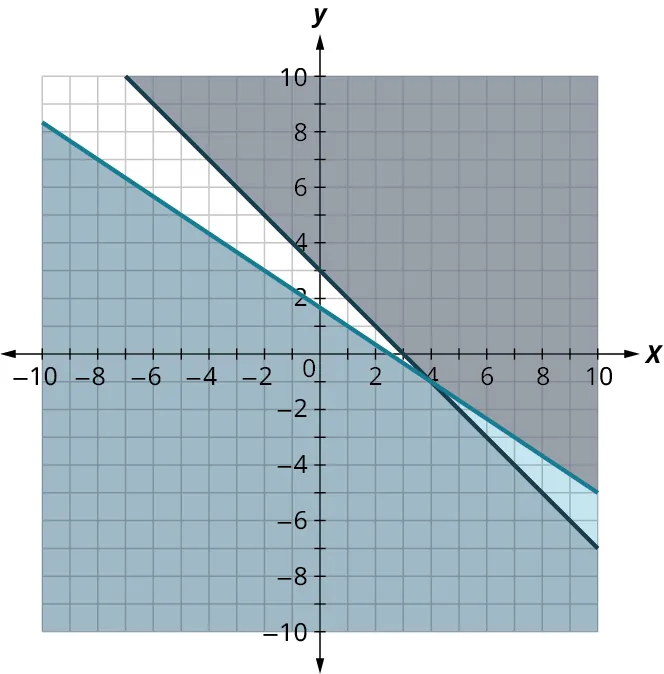2.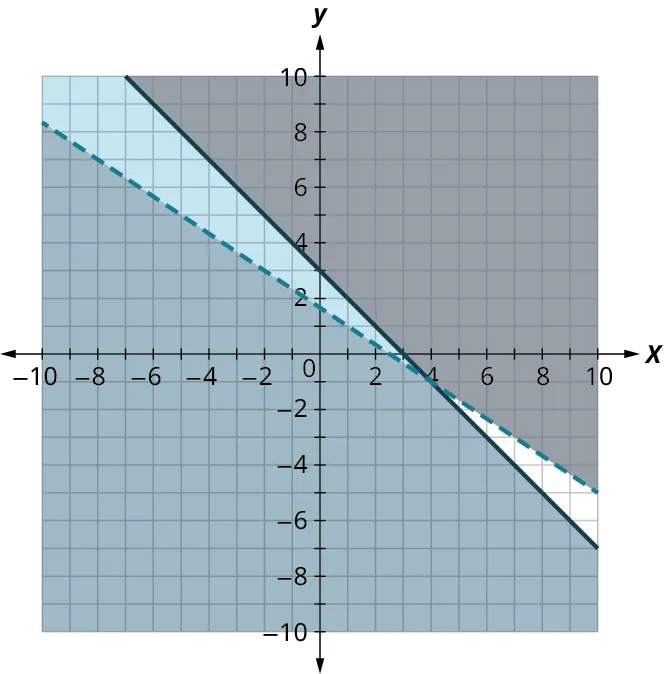3.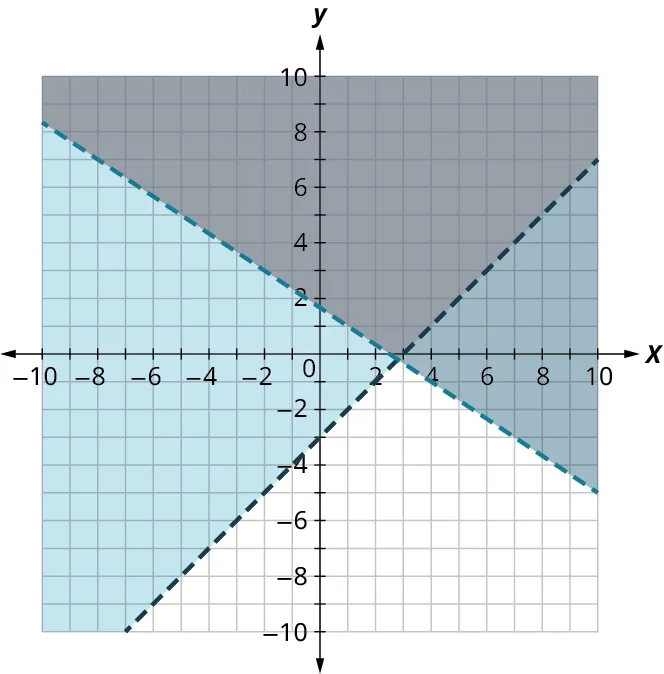4.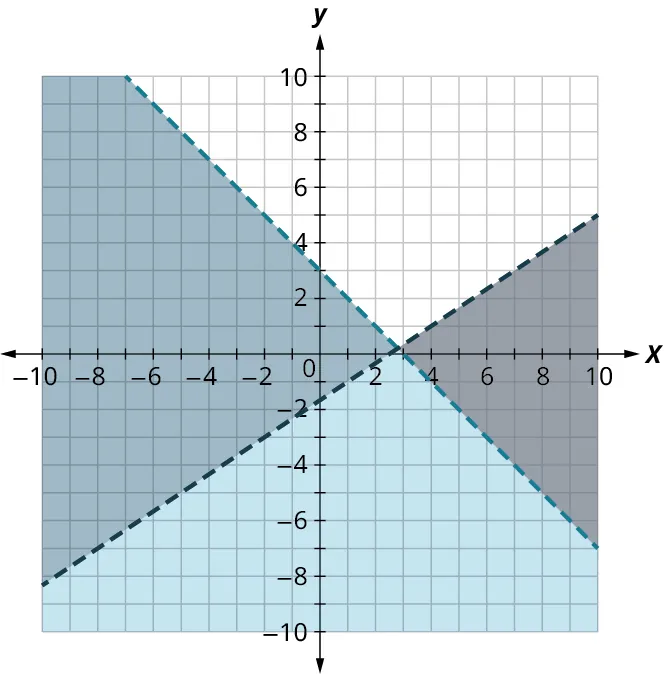5.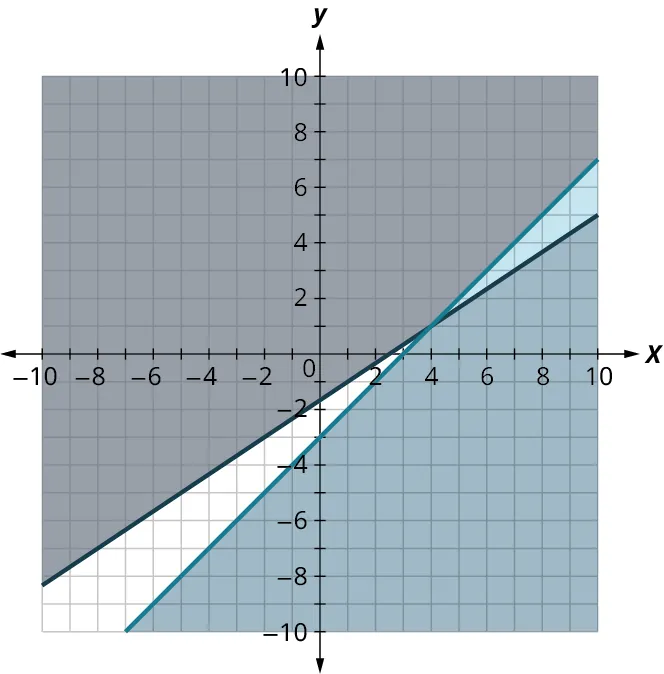76. $\left\{ \begin{array}{r} 2x - 3y \leq 5 \\ x - y \geq 3 \\ \end{array} \right.$ 1.2.3.4.5.77. $\left\{ \begin{array}{r} 2x - 3y > 5 \\ x + y < 3 \\ \end{array} \right.$ 1.2.3.4.5.78. $\left\{ \begin{array}{r} 2x + 3y > 5 \\ x + y \leq 3 \\ \end{array} \right.$ 1.2.3.4.5.79. $\left\{ \begin{array}{r} 2x + 3y \leq 5 \\ x + y \geq 3 \\ \end{array} \right.$ 1.2.3.4.5.### Section 5.10 Exercises For the following exercises, determine whether each ordered pair is a solution to the system. 1 . $\left\{ \begin{array}{l} 3x + y > 5 \\ 2x - y \leq 10 \\ \end{array} \right.$ A: $(3, –3)$ B: $(7, 1)$ 2 . $\left\{ \begin{array}{l} y < \frac{3}{2}x + 3 \\ \frac{3}{4}x - 2y < 5 \\ \end{array} \right.$ A: $(-4, -1)$ B: $(8, 3)$ 3 . $\left\{ \begin{array}{l} 4x - y < 10 \\ - 2x + 2y > - 8 \\ \end{array} \right.$ A: $(5, –2)$ B: $(–1, 3)$ 4 . $\left\{ \begin{array}{l} y > \frac{2}{3}x - 5 \\ x + \frac{1}{2}y \leq 4 \\ \end{array} \right.$ A: $(6, -4)$ B: $(3, 0)$ 5 . $\left\{ \begin{array}{l} 6x - 5y < 20 \\ - 2x + 7y > - 8 \\ \end{array} \right.$ A: $(1, –3)$ B: $(-4, 4)$ 6 . $\left\{ \begin{array}{l} 7x + 2y > 14 \\ 5x - y \leq 8 \\ \end{array} \right.$ A: $(2, 3)$ B: $(7, -1)$ For the following exercises, determine whether each ordered pair is a solution to the darkest shaded region of the graph. 7 .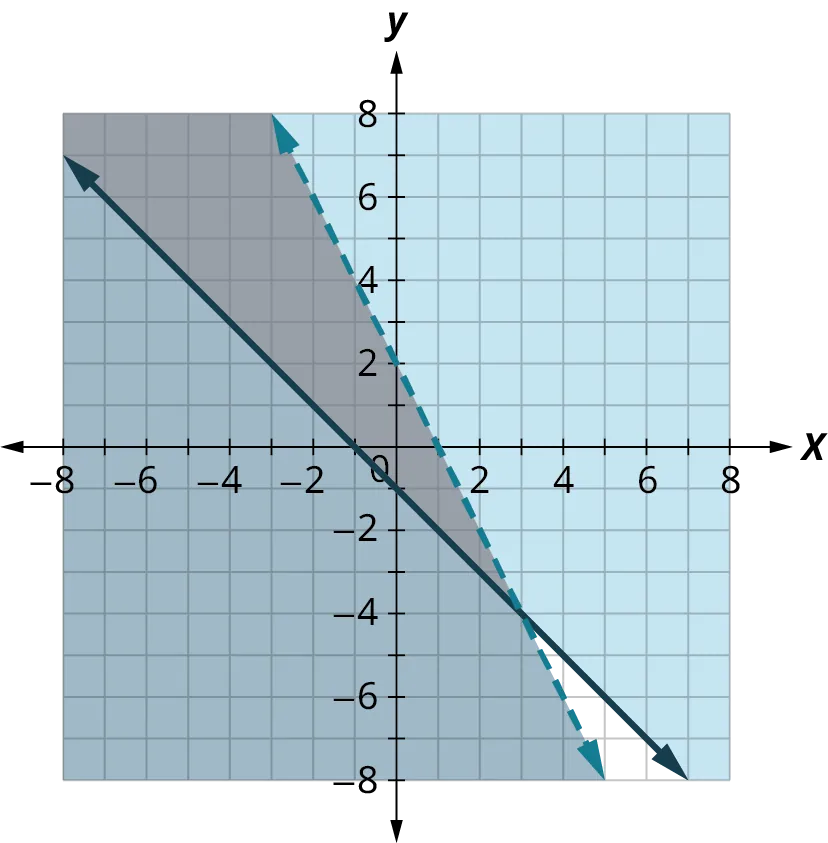A: $(0, 0)$ B: $(6, –8)$ 8 .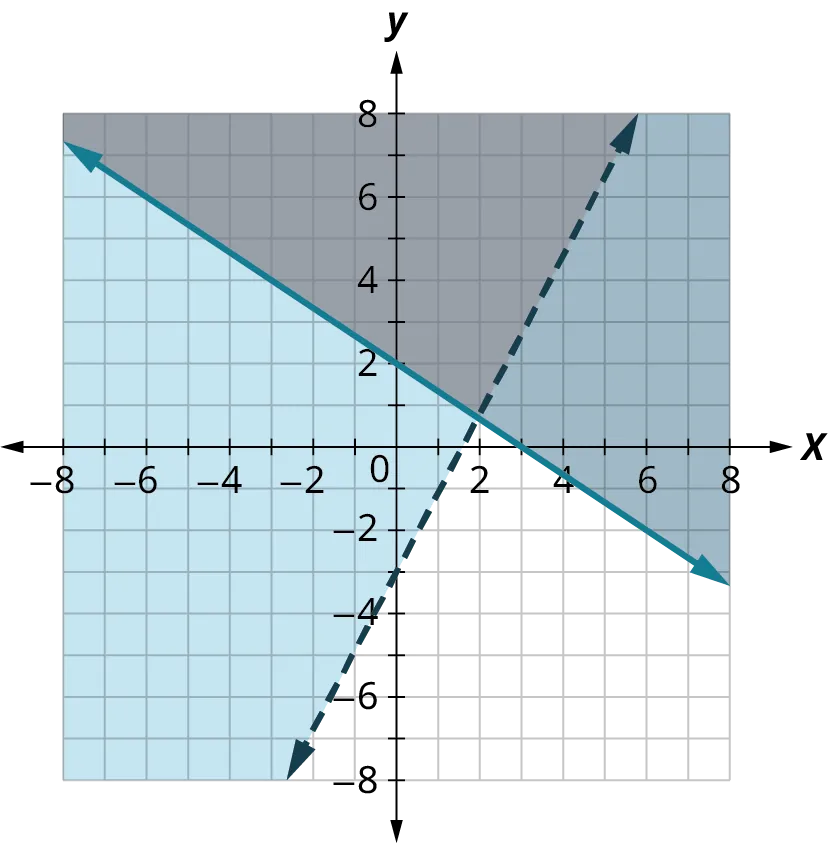A: $(0, 0)$ B: $(2, –4)$ 9 .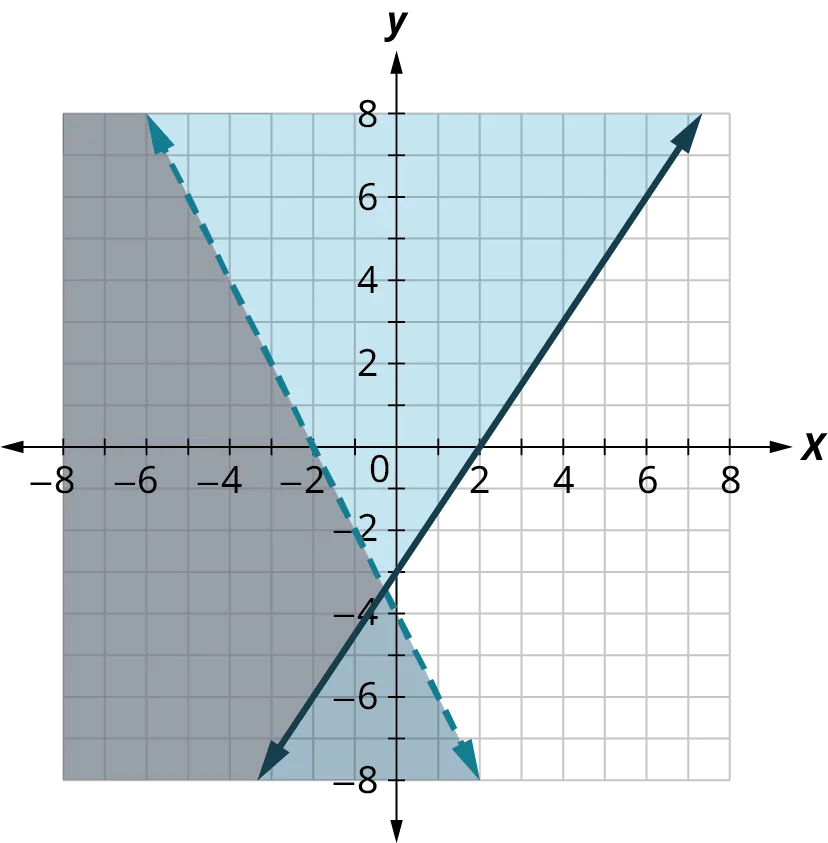A: $(0, 0)$ B: $(2, 2)$ 10 .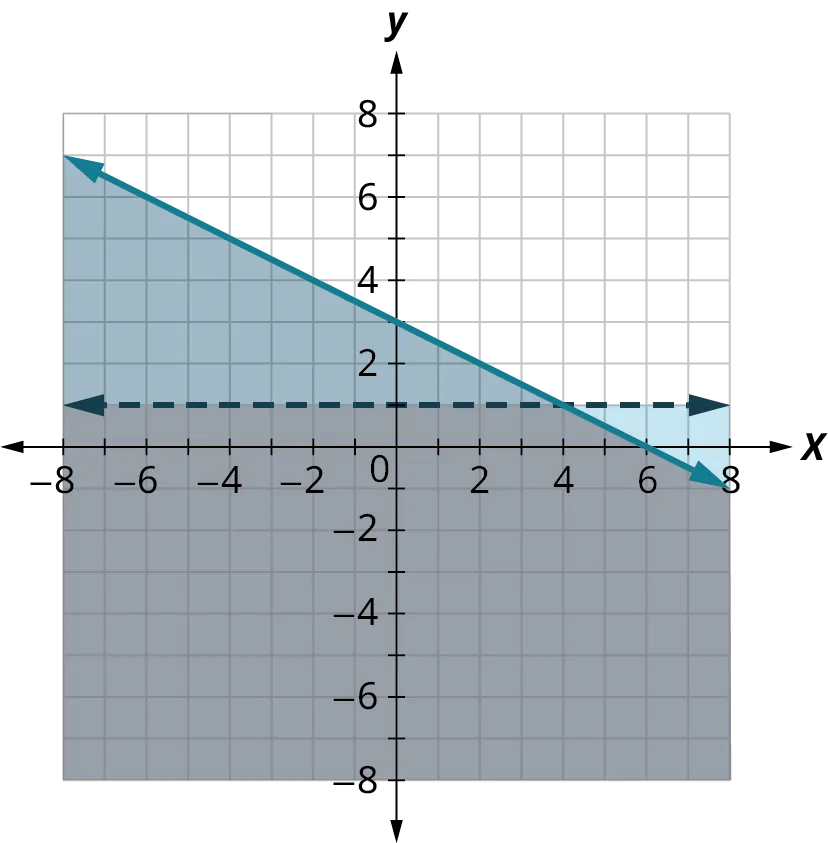A: $(0, 0)$ B: $(2, 3)$ 11 .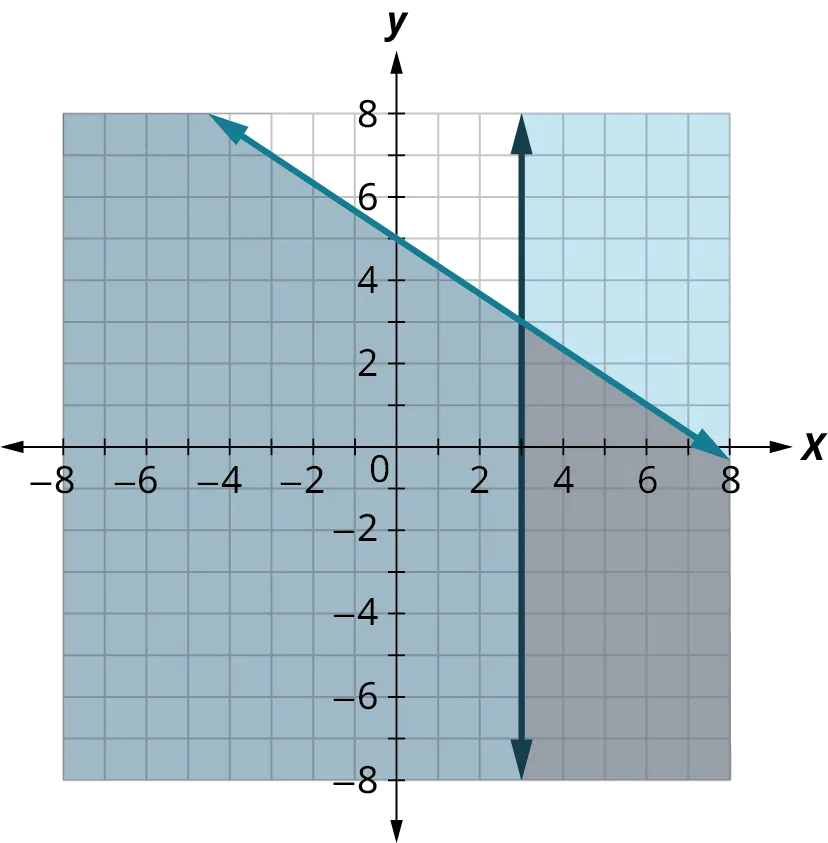A: $(0, 0)$ B: $(6, 0)$ 12 .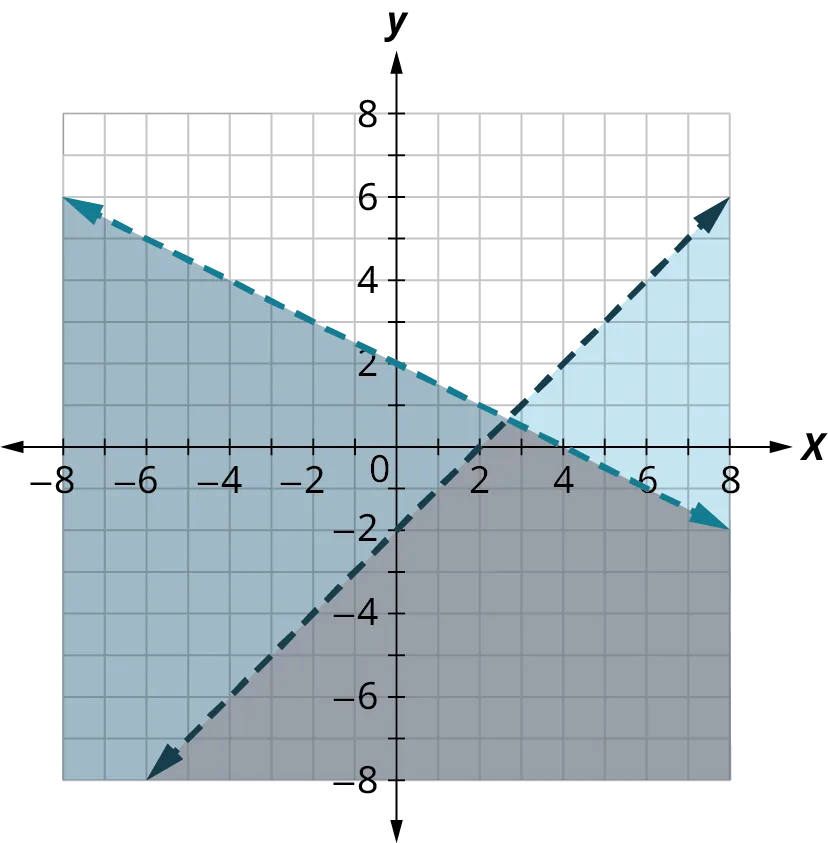A: $(0, 0)$ B: $(6, 2)$ 13 .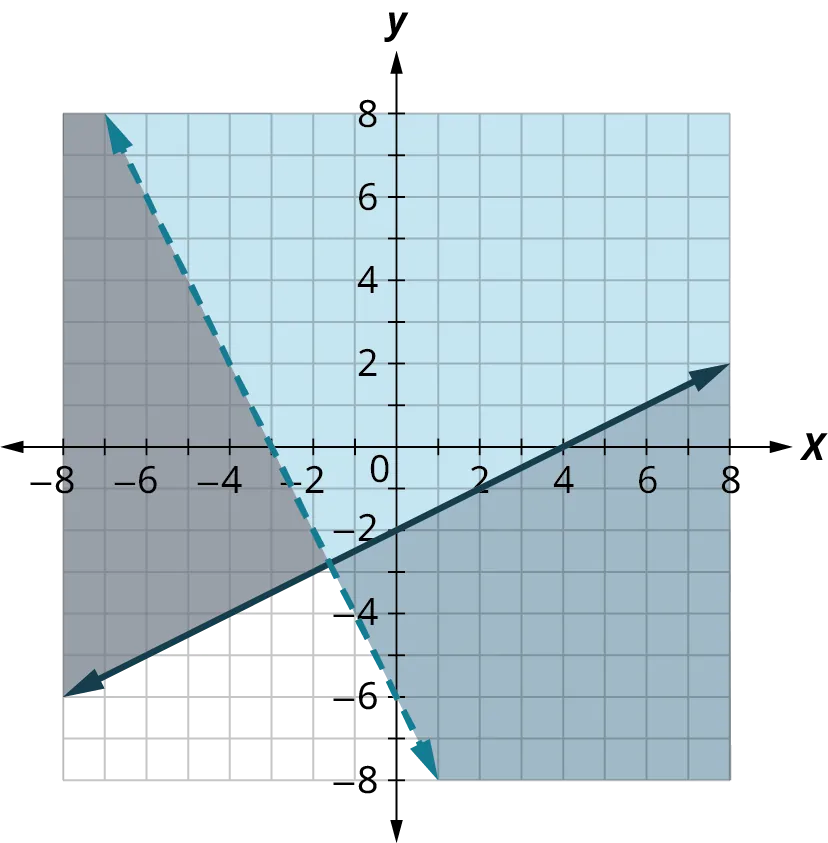A: $(0, 0)$ B: $(2, 2)$ For the following exercises, solve the systems of linear equations by graphing. 14 . $\left\{ \begin{array}{rcl}{y}&{ \leq }&{3x + 2}\\{y}&{ > }&{x - 1}\end{array} \right.$ 15 . $\left\{ \begin{array}{rcl}{y}&{ < }&{2x - 1}\\{y}&{ \leq }&{- \frac{1}{2}x + 4}\end{array} \right.$ 16 . $\left\{ \begin{array}{rcl}{x - y}&{ > }&{1}\\{y}&{ < }&{ - \frac{1}{4}x + 3}\end{array} \right.$ 17 . $\left\{ \begin{array}{rcl}{3x - y}&{ \geq }&{6}\\{y}&{ \geq }&{ - \frac{1}{2}x}\end{array} \right.$ 18 . $\left\{ \begin{array}{rcl}{2x + 4y}&{ \geq }&{8}\\{y}&{ \leq }&{\frac{3}{4}x}\end{array} \right.$ 19 . $\left\{ \begin{array}{rcl}{2x - 5y}&{ < }&{10}\\{3x + 4y}&{ \geq }&{12}\end{array} \right.$ 20 . $\left\{ \begin{array}{rcl}{2x + 2y}&{ > }&{ - 4}\\{- x + 3y}&{ \geq }&{9}\end{array} \right.$ 21 . $\left\{ \begin{array}{rcl}{x - 2y}&{ < }&{3}\\{y}&{ \leq }&{1}\end{array} \right.$ 22 . $\left\{ \begin{array}{rcl}{x - 3y}&{ > }&{4}\\{y}&{ \leq }&{ - 1}\end{array} \right.$ 23 . $\left\{ \begin{array}{rcl}{y}&{ \geq }&{ - \frac{1}{2}x - 3}\\{x}&{ \leq }&{2}\end{array} \right.$ 24 . $\left\{ \begin{array}{rcl}{x - 3y}&{ \geq }&{6}\\{y}&{ > }&{\frac{1}{3}x + 1}\end{array} \right.$ 25 . $\left\{ \begin{array}{rcl}{y}&{ \geq }&{\frac{3}{4}x - 2}\\{y}&{ < }&{2}\end{array} \right.$ 26 . $\left\{ \begin{array}{rcl}{3x - 4y}&{ < }&{8}\\{x}&{ < }&{1}\end{array} \right.$ 27 . $\left\{ \begin{array}{rcl}{- 3x + 5y}&{ > }&{10}\\{x}&{ > }&{ - 1}\end{array} \right.$ 28 . $\left\{ \begin{array}{rcl}{x}&{ \geq }&{3}\\{y}&{ \leq }&{2}\end{array} \right.$ 29 . $\left\{ \begin{array}{rcl}{x}&{ \leq }&{ - 1}\\{y}&{ \geq }&{3}\end{array} \right.$ 30 . $\left\{ \begin{array}{rcl}{2x + 4y}&{ > }&{4}\\{y}&{ \leq }&{ - \frac{1}{2}x - 2}\end{array} \right.$ For the following exercises, translate to a system of inequalities and solve. A gardener does not want to spend more than$50 on bags of fertilizer and peat moss for their garden. Fertilizer costs $2 a bag and peat moss costs$5 a bag. The gardener’s van can hold at most 20 bags.
31 .
Write a system of inequalities to model this situation.
32 .
Graph the system.
33 .
Can they buy 15 bags of fertilizer and 4 bags of peat moss?
34 .
Can they buy 10 bags of fertilizer and 10 bags of peat moss?
For the following exercises, translate to a system of inequalities and solve.
A student is studying for their final exams in chemistry and algebra. They only have 24 hours to study, and it will take them at least 3 times as long to study for algebra than chemistry.
35 .
Write a system of inequalities to model this situation.
36 .
Graph the system.
37 .
Can they spend 4 hours on chemistry and 20 hours on algebra?
38 .
Can they spend 6 hours on chemistry and 18 hours on algebra?
For the following exercises, translate to a system of inequalities and solve.
Mara is attempting to build muscle mass. To do this, she needs to eat an additional 80 grams of protein or more in a day. A bottle of protein water costs $3.20 and a protein bar costs$1.75. The protein water supplies 27 grams of protein and the bar supplies 16 grams. Let $w$ be the number of water bottles Mara can buy, and let $b$ be the number of protein bars she can buy. If Mara has $10 dollars to spend: 39 . Write a system of inequalities to model this situation. 40 . Graph the system. 41 . Could she buy 3 bottles of protein water and 1 protein bar? 42 . Could she buy no bottles of protein water and 5 protein bars? For the following exercises, translate to a system of inequalities and solve. Mark is increasing his exercise routine by running and walking at least 4 miles each day. His goal is to burn a minimum of 1,500 calories from this exercise. Walking burns 270 calories/mile and running burns 650 calories/mile. 43 . Write a system of inequalities to model this situation. 44 . Graph the system. 45 . Could he meet his goal by walking 3 miles and running 1 mile? 46 . Could he meet his goal by walking 2 miles and running 2 miles? For the following exercises, translate to a system of inequalities and solve. Tension needs to eat at least an extra 1,000 calories a day to prepare for running a marathon. He has only$25 to spend on the extra food he needs and will spend it on $0.75 donuts, which have 360 calories each, and$2 energy drinks, which have 110 calories.
47 .
Write a system of inequalities that models this situation.
48 .
Graph the system.
49 .
Can he buy 8 donuts and 4 energy drinks and satisfy his caloric needs?
50 .
Can he buy 1 donut and 3 energy drinks and satisfy his caloric needs?
Order a print copy

As an Amazon Associate we earn from qualifying purchases.